## RD Sharma Class 10 Solutions Chapter 1 Real Numbers Ex 1.2

These Solutions are part of RD Sharma Class 10 Solutions. Here we have given RD Sharma Class 10 Solutions Chapter 1 Real Numbers Ex 1.2

Other Exercises

Question 1.
Define H.C.F. of two positive integers and find the H.C.F. of the following pairs of numbers.
(i) 32 and 54
(ii) 18 and 24
(iii) 70 and 30
(iv) 56 and 88
(v) 475 and 495
(vi) 75 and 243
(vii) 240 and 6552
(viii) 155 and 1385
(ix) 100 and 190
(x) 105 and 120
Solution:
Definition : The greatest among the common divisor of two or more integers is the Greatest Common Divisor (G.C.D.) or Highest Common Factor (H.C.F.) of the given integers.
(i) HC.F. of 32 and 54
Factors 32 = 1, 2, 4, 8, 16, 32
and factors of 54 = 1, 2, 3, 6, 9, 18, 27, 54
H.C.F. = 2
(ii) H.C.F. of 18 and 24
Factors of 18 = 1, 2, 3, 6, 9, 18
and factors of 24 = 1, 2, 3, 4, 6, 8, 12, 24
Highest common factor = 6
H.C.F. = 6
(iii) H.C.F. of 70 and 30
Factors of 70 = 1, 2, 5, 7, 10, 14, 35, 70
and factors of 30 = 1, 2, 3, 5, 6, 10, 15, 30
H.C.F. = 10
(iv) H.C.F. of 56 and 88
Factors of 56 = 1, 2, 4, 7, 8, 14, 28, 56
and factors of 88 = 1, 2, 4, 8, 11, 22, 44, 88
H.C.F. = 8
(v) H.C.F. of 475 and 495
Factors of 475 = 1, 5, 25, 19, 95, 475
and factors of 495 = 1, 3, 5, 9, 11, 15, 33, 45, 55, 99, 165, 495
H.C.F. = 5
(vi) H.C.F. of 75 and 243
Factors of 75 = 1, 3, 5, 15, 25, 75
Factors of 243 = 1, 3, 9, 27, 81, 243
H.C.F. = 3
(vii) H.C.F. of 240 and 6552
Factors of 240 = 1, 2, 3, 4, 5, 6, 8, 10, 12, 15, 16, 20, 24, 48, 60, 80, 120, 240
Factors of 6552 = 1, 2, 3, 4, 6, 7, 8, 9, 12, 13, 14, 18, 21, 24, 26, 28, 36, 39, 42, 52, 56, 63, 72, 91, 104, 117, 126, 156, 168, 182, 234,252, 273, 312, 364, 488, 504, 546, 728, 819, 936, 1092, 1638, 2184, 3276, 6552
H.C.F. = 24
(viii) H.C.F. of 155 and 1385
Factors of 155 = 1, 5, 31, 155
Factors of 1385 = 1, 5, 277, 1385
H.C.F. = 5
(ix) 100 and 190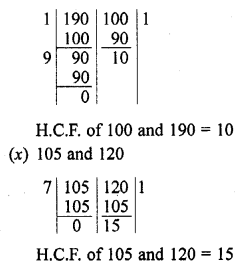Question 2.
Use Euclid’s division algorithm to find the H.C.F. of
(i) 135 and 225
(ii) 196 and 38220
(iii) 867 and 255
(iv) 184, 230 and 276
(v) 136,170 and 255
Solution:
(i) H.C.F. of 135 and 225
135 < 225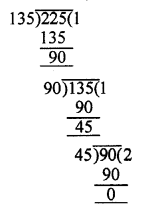225 = 135 x 1 + 90
135 = 90 x 1 +45
45 = 45 x 2 + 0
Last remainder = 0
and last divisor = 45
H.C.F. = 45
(ii) H.C.F. of 196 and 38220
196 < 38220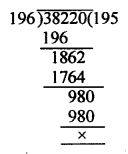38220 = 196 x 195 + 0
Last remainder = 0
and last divisor = 196
H.C.F. = 196
(iii) H.C.F. 867 and 255
255 < 867867 = 255 x 3 + 102
255 = 102 x 2 + 51
102 = 51 x 2 + 0
Last remainder = 0
and last divisor = 51
H.C.F. = 51
(iv) H.C.F. of 184, 230 and 276
Let us find the highest common factor (H.C.F.) of 184 and 230Hence, H.C.F. of 184 and 230 = 46
Now, find the H.C.F. of 276 and 46Hence, H.C.F. of 276 and 46 = 46
Required H.C.F. of 184, 230 and 276 = 46
(v) H.C.F. of 136, 170 and 255
Let us find the highest common factor (H.C.F.) of 136 and 70Hence, H.C.F. of 136 and 170 = 34
Now, find the H.C.F. of 34 and 255Hence, highest common factor of 34 and 255 = 17
Required H.C.F. of 136, 170 and 255 = 17

Question 3.
Find the H.C.F. of the following pairs of integers and express it as a linear combinations of them.
(i) 963 and 657
(ii) 592 and 252
(iii) 506 and 1155
(iv) 1288 and 575
Solution:
(i) 963 and 657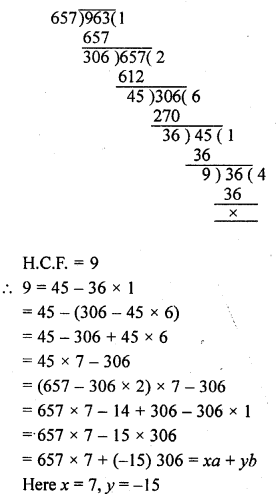(ii) HCF of 592 and 25276x + 88y
Where x = 7, y = -6
(iii) 506 and 1155
H.C.F. = 11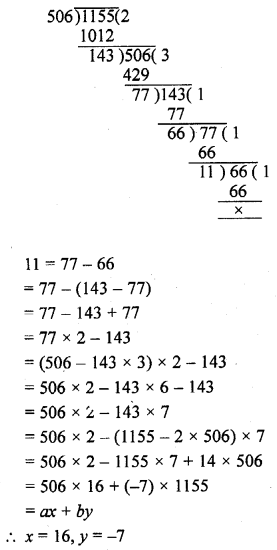(iv) 1288 and 575
H.C.F. = 23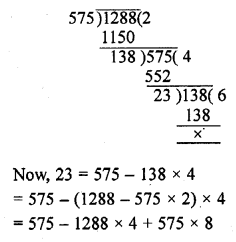= 575 x 9 + 1288 x (-4)
= ax + by
x = 9, y = -4

Question 4.
Find the largest number which divides 615 and 963 leaving remainder 6 in each case.
Solution:
The given numbers are 615 and 963
Remainder in each case = 6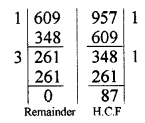615 – 6 = 609 and 963 – 6 = 957 are divisible by the required number which is the H.C.F. of 609 and 957 = 87
Hence the required largest number = 87

Question 5.
If the H.C.F. of 408 and 1032 is expressible in the form 1032m – 408 x 5, find m.
Solution:
408, 1032
H.C.F. = 24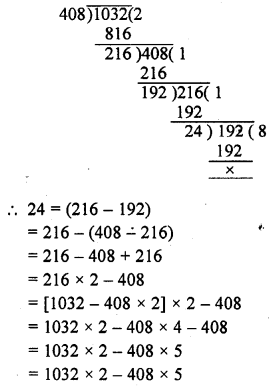Which is in the form of 1032m – 408 x 5 comparing, we get m = 2

Question 6.
If the H.C.F. of 657 and 963 is expressible in the form 657x + 963 x (-15), find x.
Solution:
657 and 963
H.C.F. = 9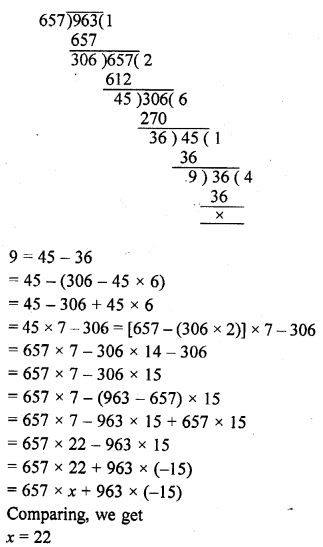Question 7.
An army contingent of 616 members is to march behind an army band of 32 members in a parade. The two groups are to march in the same number of columns. What is the maximum number of columns in which they can march ?
Solution:
The required number of columns will be the H.C.F. of 616 and 32Using Euclid’s division
We get H.C.F. = 4
Number of columns = 4

Question 8.
A merchant has 120 litres of oil of one kind, 180 litres of another kind and 240 litres of third kind. He wants to sell the oil by filling the three kinds of oil in tins of equal capacity. What should be the greatest capacity of such a tin ?
Solution:
Quantity of oil of one kind =120l
and quantity of second kind = 180l
and third kind of oil = 240l
Maximum capacity of oil in each tin = H.C.F. of 120l, 180l and 240l
H.C.F. of 120 and 180 = 60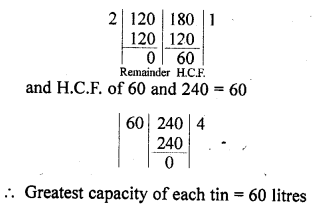Question 9.
During a sale, colour pencils were being sold in packs of 24 each and crayons in packs of 32 each. If you want full packs of both and the same number of pencils and crayons, how many of each would you need to buy ?
Solution:
Number of pencils in each pack = 24
and number of crayons pack = 32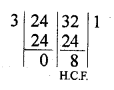Highest number of pencils and crayons in packs will be = H.C.F. of 24 and 32 = 8
Number of pencil’s pack = $$\frac { 24 }{ 8 }$$ = 3
and number of crayon’s pack = $$\frac { 32 }{ 8 }$$ = 4

Question 10.
144 cartons of Coke Cans and 90 Cartons of Pepsi Cans, are to be stacked in a Canteen. If each stack is of the same height and is to contain cartons of the same drink what would be the greatest number of cartons each stack would have?
Solution:
Number of Coke Cans Cartons = 144
and number Pepsi Cartons = 90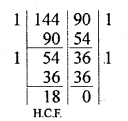Required greatest number of cartons of each = H.C.F. of 144 and 90 = 18

Question 11.
Find the greatest number which divides 285 and 1249 leaving remainders 9 and 7 respectively.
Solution:
The given numbers are 285 and 1249 Remainder are 9 and 7 respectively
285 – 9 = 276
and 1249 – 7 = 1242 are divisible by required number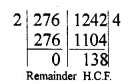Required number = H.C.F. of 276 and 1242
Now, H.C.F. of 276 and 1242 = 138
Required number = 138

Question 12.
Find the largest number which exactly divides 280 and 1245 leaving remainders 4 and 3, respectively.
Solution:
The given numbers are 280 and 1245 Remainder are 4 and 3 respectively
280 – 4 = 276 and 1245 – 3 = 1242 are divisible by a number
The required number = H.C.F. of 276 and 1242H.C.F. of 276 and 1242 = 138
Hence required number =138

Question 13.
What is the largest number that divides 626, 3127 and 15628 and leaves remainders of 1, 2 and 3 respectively.
Solution:
Given numbers are 626, 3127 and 15628 and remainders are 1, 2 and 3 respectively
626 – 1 = 6253127 – 2 = 3125 and 15628 – 3 = 15625 are divisible by a required greatest numberThe greatest number will be the H.C.F. of 625, 3125 and 15625
H.C.F. of 625 and 3125 = 625
and H.C.F. of 625 and 15625 = 625
The required number = 625

Question 14.
Find the greatest numbers that will divide 445, 572 and 699 leaving remainders 4, 5 and 6 respectively.
Solution:
Given numbers are 445, 572 and 699
and remainders are 4, 5, 6 respectively
445 – 4 = 441
572 – 5 = 567699 – 6 = 693 are exactly divisible by a certain number which is the H.C.F. of these numbers
H.C.F. of 441 and 567 = 63
and H.C.F. of 63 and 693 = 63The required number = 63

Question 15.
Find the greatest number which divides 2011 and 2623 leaving remainders 9 and 5 respectively.
Solution:
The given numbers are 2011 are 2623 and remainders are 9 and 5 respectively
2011 – 9 = 2002 and 2623 – 5 = 2618 are divisible by a greatest number which is the H.C.F. of 2002 and 2618H.C.F. = 2002 and 2618 = 154
The required number= 154

Question 16.
Using Euclid’s division algorithm, find the largest number that divides 1251, 9377 and 15628 leaving remainders 1, 2 and 3 respectively. [NCERT Exemplar]
Solution:
Since, 1,2 and 3 are the remainders of 1251, 9377 and 15628, respectively.
Thus, after subtracting these remainders from the numbers.
We have the numbers, 1251 – 1 = 1250, 9377 – 2 = 9375 and 15628 – 3 = 15625
which is divisible by the required number.
Now, required number = HCF of 1250, 9375 and 15625 [for the largest number]
By Euclid’s division algorithm,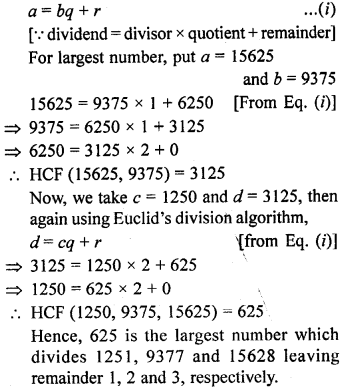Question 17.
Two brands of chocolates are available in packs of 24 and 15 respectively. If I need to buy an equal number of chocolates of both kinds, what is the least number of boxes of each kind I would need to buy ?
Solution:
Number of chocolates of first kind = 24 and of second kind = 15
Number of chocolates to be bought equally of both kinds = H.C.F. of 24 and 15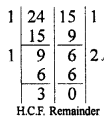= 3 chocolates
Least number of boxes of first kind = $$\frac { 24 }{ 3 }$$ = 8
and of second kind = $$\frac { 15 }{ 3 }$$ = 5

Question 18.
A mason has to fit a bathroom with square marble tiles of the largest possible size. The size of the bathroom is 10 ft. by 8 ft. What would be the size in inches of the tile required that has to be cut and how many such tiles are required ?
Solution:
Size of bathroom = 10 ft. x 8 ft.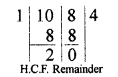Largest size of tile = H.C.F. of 10 ft. and 8 ft. = 2 ft.
= 2 x 12 = 24 inches (1 ft. = 12 inches)

Question 19.
15 pastries and 12 biscuit packets have been donated for a school fete. These are to be packed in several smaller identical boxes with the same number of pastries and biscuit packets in each. How many biscuit packets and how many pastries will each box contain ?
Solution:
Number of pastries = 15
and number of biscuit packets =12
The number of pastries and pack of biscuits to be packed in smaller identical boxes
H.C.F. of 15 and 12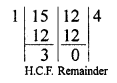H.C.F. = 3
Each box will contain = $$\frac { 15 }{ 3 }$$ pastries and $$\frac { 12 }{ 3 }$$ pack of biscuits
= 5 pastries and 4 pack of biscuits

Question 20.
105 goats, 140 donkeys and 175 cows have to be taken across a river. There is only one boat which will have to make many trips in order to do so. The lazy boatman has his own conditions for transporting them. He insists that he will take the same number of animals in every trip and they have to be of the same kind. He will naturally like to take the largest possible number each time. Can you tell how many animals went in each trip ?
Solution:
The required number of animals will be the H.C.F. of 105 goats, 140 donkeys, 175 cows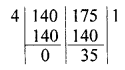H.C.F. of 175 and 140 = 35
and H.C.F. of 35 and 105 = 35The required number of animals = 35

Question 21.
The length, breadth and height of a room are 8m 25 cm, 6 m 75 cm and 4 m 50 cm respectively. Determine the longest rod which can measure the three dimensions of the room exactly.
Solution:
Length = 8m 25 cm = 825 cm
Breadth = 6 m 75 cm = 675 cm
Height = 4 m 50 cm = 450 cmThe required measure will be the H.C.F. of these three dimensions
H.C.F. of 825 and 675 = 75
and H.C.F. of 75 and 450 = 75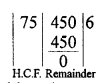The required length = 75 cm

Question 22.
Express the H.C.F. of 468 and 222 as 468x + 222y where x, y are integers in two different ways.
Solution:
468 and 222
H.C.F. = 6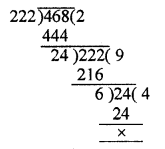6 = (222 – 24 x 9)
= 222 – (468 – 222 x 2) x 9
= 222 – 468 x 9 + 222 x 18
= 222 x 19 + 468 x (-9)
= 468 (9) + 222 x 19
Which is in the form of 468x + 222y
Similarly we can write it in the following form also
6 = 468 x 213 +222 x (-449)

Hope given RD Sharma Class 10 Solutions Chapter 1 Real Numbers Ex 1.2 are helpful to complete your math homework.

If you have any doubts, please comment below. Learn Insta try to provide online math tutoring for you.